## research papersBIOLOGICALCRYSTALLOGRAPHY
ISSN: 1399-0047
Volume 58| Part 11| November 2002| Pages 1915-1923

## Computational analysis of crystallization trials

aGenomics Institute of the Novartis Research Foundation, 10675 John Jay Hopkins Drive, La Jolla CA 92121, USA, and bNovartis Pharma AG, CH-4002 Basel, Switzerland
*Correspondence e-mail: spraggon@gnf.org

(Received 7 May 2002; accepted 16 September 2002)

A system for the automatic categorization of the results of crystallization experiments generated by robotic screening is presented. Images from robotically generated crystallization screens are taken at preset time intervals and analyzed by the computer program Crystal Experiment Evaluation Program (CEEP). This program attempts to automatically categorize the individual crystal experiments into a number of simple classes ranging from clear drop to mountable crystal. The algorithm first selects features from the images via edge detection and texture analysis. Classification is achieved via a self-organizing neural net generated from a set of hand-classified images used as a training set. New images are then classified according to this neural net. It is demonstrated that incorporation of time-series information may enhance the accuracy of classification. Preliminary results from the screening of the proteome of Thermotoga maritima are presented showing the utility of the system.

### 1. Introduction

The structural genomics initiative requires many tools to automate procedures normally performed manually. Not least of these processes is the detection of crystals within crystallization trials. Modern robotic crystallization systems provide the ability to screen 10–100 000 crystallization conditions per day (Stevens, 2000). Added to this complexity, multiple images of a crystallization trial may be taken at distinct time points, amounting to a large number of images to evaluate. To deal with the large number of images obtained, a quick computational method must be designed to filter the number of images down to a manageable number for visual inspection whilst maintaining a high success rate in identifying crystal-containing drops. In the past, such methods have been applied to crystal images; for example, Zuk & Ward (1991) used the Hough transform (Hough, 1962) to identify crystal edges. Such methods are still applicable today. In addition to crystal identification, it would be useful to score or annotate the individual non-crystallizing experiments' to provide valuable information as to where to proceed in subsequent trials. Such data would also provide a valuable metric for the design of more efficient screens.

Image analysis is a vast computational field whose algorithms generally progress through various stages of segmentation of an image into its interesting features' followed by classification of these features into various types via pattern matching or other algorithms. Besides the noise introduced by digitizing an image, the problem with most of these techniques is determining the correct relative weighting of each of the extracted features to provide a definitive classification.

In general, humans have little difficulty identifying crystals or potential crystals. Although the exact mechanism whereby any pattern is identified by visual inspection remains to be determined, it is simple to identify some key features that set crystals apart from other objects. Straight edges, flat facets, symmetry and objects that are closed are only a few of the features that can be used to describe crystals. All of these features' may be violated, but are on the whole true. The key is to identify the correct features to choose and then define the set of values of these features that are compatible with a crystal' or other pattern in a drop.

One such method for solving this problem is the use of self-organizing neural nets (Kohonen maps; Kohonen, 1982), which map a many-dimensional feature vector onto a two-dimensional map of artificial neurons. To implement such a procedure for crystallization experiments, a computer program, Crystal Experiment Evaluation Program (CEEP), is being developed. In CEEP, a number of features are extracted from a set of images with known classification and are then trained in an unsupervised way such that like images with similar features cluster together on a plane of neurons. Once this planar map has been trained, each node, consisting of one neuron in the plane, may be assigned to an individual classification. Subsequent annotation of unclassified images is then carried out by extracting features from an image and then finding the node that this image lands on in the classification map (Fig. 1).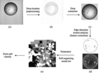Figure 1 Schematic of Crystal Experiment Evaluation Program. (a) Image is located after generation by imager; (b) test for presence of drop – if no drop classify as 0; (c) extract the drop from the superfluous material around it; (d) extract feature vector (pattern); (e) present the pattern to the neural net and classify.

Here, as part of the Joint Center for Structural Genomics, we present a preliminary analysis of crystallization trials with CEEP from the crystallization results of expression of the entire proteome of Thermotoga maritima, one of the structural genomics initiatives funded by the NIH.

### 2. Methods

#### 2.1. Crystallization

All proteins were crystallized using the sitting-drop vapor-diffusion method at 293 K in custom 96-well plates (manufactured by Greiner Inc.) using sparse-matrix screens from Hampton Research and Emerald Biostructures and a custom robotics system. Typically, total drop size was 100 nl using 50 nl of protein and 50 nl precipitant, giving a total drop diameter of approximately 0.5–1 mm.

#### 2.2. Image acquisition

Images were taken between 2 and 4 h after set-up and then at 7 and 28 d intervals with an Optimag 1700 (Veeco-Optimag San Diego, CA, USA) imaging system equipped with a 5× magnification objective fixed-focus microscope. The system is capable of taking 96 (one plate) 256 gray-level images (Microsoft bitmap format) per 60 s (Fig. 2).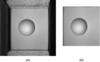Figure 2 Typical bitmap generated by Optimag 1700 machine. (a) Unextracted full 1 Mb bitmap image (1024 × 1024 pixels = 1 Mb), (b) crystallization shelf extracted using correlation with a 1.92 × 2.0 mm box (700 × 750 pixels = 0.5 Mb).

#### 2.3. Preprocessing

The image of the shelf on which the crystallization drop sits is automatically extracted by matching a 1.9 × 2.0 mm box with the shelf edges (Fig. 1). This reduces the image to be analyzed from 1024 × 1024 pixels (1 Mb) to 700 × 750 pixels (0.5 Mb). As a first step, the grayscale gradients on the image are calculated (described in detail below). Examination of these gradients is performed to determine how well focused the image is. A well focused image should have sharp edges giving rise to large gradients, while a badly focused (blurred) image has smoother edges and lower gradients. The amount of blurring can be expressed as in terms of convolution of a sharp in-focus image with a two-dimensional Gaussian function of a given σ value. The larger the σ value, the more blurred the imaged and the lower the gradients. Examination of a large number of images has shown that σ values less than 2.5 imply a generally well focused image. Values between 2.5 and 4.0 are noticeably out of focus. Values of σ greater than 4.0 usually mean that there is no drop on the shelf and greater than 5.0 usually imply serious condensation on the tape above the drop, masking the drop and its contents.

The next step is to locate the crystallization drop on the shelf. The edge-detection method of Canny (1986) as implemented by Heath et al. (1998) is used to convert the eight bit per pixel gray-level image to a one bit per pixel edge image. Firstly, the image is convoluted with a Gaussian function, i.e. blurred. This is necessary to produce smooth edges. A Gaussian function with σ = 2.0 was empirically found to produce sufficient blurring without being too computationally expensive. Next, the gradients in both the x (GX) and y directions (GY) are determined by substituting the gray-level at each point in the image (GLx,y) with GLx + 1, y − GLx − 1, y for the x-­direction gradient and GLx, y + 1 − GLx, y − 1 for that in the y direction. This is equivalent to convolution with the one-dimensional filter [+1 0 −1]. The magnitude of the gradient is then calculated by combining the x and y one-dimensional gradients such that Gx,y = (GXx,y2 + GYx,y2)1/2. Non-maximal suppression is then performed on the gradient image, which traces along ridges of highest G, accepting as edges only gradients higher than some user-defined threshold (Thigh) (Fig. 3b). These edges are then extended along gradients higher than a second user-defined threshold (Tlow). The values of these thresholds are compromises that interesting' edges, e.g. drop edges and crystal edges, are found, but uninteresting' edges, e.g. imperfections in the plastic of the shelf, are ignored.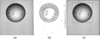Figure 3 Location of the drop boundary. (a) Original image, (b) after edge detection. The drop boundary has been identified as the largest curved edge in the image. (c) A box is fitted about the drop for extraction. The larger box around the edge of the image indicates the edges of the shelf on which the drop sits. The walls around the shelf (outside the large box) are ignored in the image analysis.

The drop edge is assumed to be the largest curved edge on the image. Further restriction on the curvature of the edge, the possible radius of the drop and roundness' (deviation from a perfect circle), again defined by the user, aid in weeding out incorrect edges. For very weak drops, i.e. those with low-contrast edges, more than one edge along the drop may be found. These are all considered together in defining the drop boundary. A perfect circle is then fit to the edge to define the crystallization drop (Fig. 3).

#### 2.4. Feature extraction

##### 2.4.1. Edges

Edges within the drop are identified using the same procedure as for finding the drop edges, except that different threshold levels may be selected (Fig. 4). Edges are then defined in terms of their location, length (number of connected pixels), closedness' (distance between the first and last pixels relative to the total length of the edge) and compactness (ratio of the maximum distance of any pixel from the center to the average distance of all pixels from the center). The area enclosed by the edge is calculated by a formula derived from the relations between a circle's circumference (c = 2πr) and its area (A = πr2) and takes into account its nearness to a closed circle (closedness) and its deviation from a circle (compactness),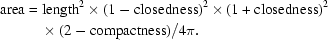Hence, for a perfect circle (closedness = 0, compactness = 1) the area in pixels is c2/4π, as it should be, while for a straight line (closedness = 1, compactness = 2) the area is zero.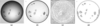Figure 4 Edge detection. (a) Original image (extracted drop from Fig. 2). (b) Image after gradient calculation (see §2.4.1). (c) All edges found after non-maximal suppression. (d) Edges found above threshold Thigh and extended if above Tlow.

Another potentially meaningful parameter is the length of any straight edge, one of the characteristics of a crystal and the parameter examined in detail by Zuk & Ward (1991). The Hough transform (Hough, 1962) is used to locate straight lines on the edge image. The Hough transform recasts pixel coordinates in xy space into polar coordinates ρ and θ (Fig. 5),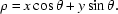ρ is then calculated for θ varying between 0 and 180° (after which it repeats itself) in 1° steps. Pixels that are collinear all intersect at the point (ρ, θ) that defines the line. The length of the line is the number of intersecting curves. While performing the Hough transform on the entire image would be computationally expensive, if it is only performed for the pixels of the individual edge groups, it becomes tractable, especially if sine and cosine look-up tables are used. Since a single long straight edge is more indicative of a nice crystal than a large number of short edges, the square of the length of the line is used to overweight long straight edges.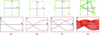Figure 5 Simple Hough transforms. Above, x, y image space; below, ρ, θ parameter space. (a) A single point. (b) Two points; the intersection in the Hough transform defines the line between the points. (c) Three points. Three intersections define the three lines between the points. (d) A triangle. The lines in the Hough transform cluster at three points that define the three lines forming the sides of the triangle.

From the edge analysis six parameters are returned: the number of individual edges found, the total area enclosed by edges, the total straight-edge length of all edges, the maximum area enclosed by a single edge, the maximum straight-edge score from a single edge and the maximum score of a single edge (straight-edge score × enclosed area) (Table 1).

 Table 1Currently used features
 Summary of commonly used features in the feature vector; features of type edge are always calculated, whilst those of gray-level co-occurrence may be calculated in five individual directions with ten possible window sizes.
Feature Type Options
No. of groups Edge
Total area of groups Edge
Summed edge length Edge
Largest group area Edge
Largest edge length Edge
Largest group area × largest group length Edge
Entropy Gray-level co-occurrence Isotropic, anisotropic four directions, ten window sizes
Homogeneity Gray-level co-occurrence Isotropic, anisotropic four directions, ten window sizes
Energy Gray-level co-occurrence Isotropic, anisotropic four directions, ten window sizes
Contrast Gray-level co-occurrence Isotropic, anisotropic four directions, ten window sizes
Standard deviation Gray-level co-occurrence Isotropic, anisotropic four directions, ten window sizes
##### 2.4.2. Textures

Texture has long been used as a segmentation device in the computational analysis of images (Haralick et al., 1973). The basic theory behind this is the construction of gray-level co-occurrence matrices. These are defined as the probability that a pixel located at position (xij) is the same as that located at distance d pixels away in a particular direction. Four directions, horizontal, vertical and the two diagonals as well as an isotropic all direction parameter' can be calculated for a distance d pixels away. Although using histograms to calculate the value of a function per pixel greatly increases the speed of the algorithm (Unser, 1986), the computational price grows rapidly as the window size increases. In CEEP, five main textures can be calculated, entropy, energy, homogeneity, root-mean-squared deviation (r.m.s.d.) and contrast, with up to four different directions and ten different distances d (Table 1; Fig. 6). Global parameters can be derived from these textures, extracted as the mean (or r.m.s.d.) value of the transformed image. Otherwise, the transformed images may be segmented and parameters such as those described for edge detection extracted (Table 1; Fig. 6).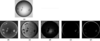Figure 6 Texture transformation on an image. Images were generated from a gray-level co-occurance matrix with isotropic direction and a pixel size d of six pixels; pij is the probability that a pixel at position ij will have the same value as one a distance d pixels away. (a) Original untransformed image; (b) entropy,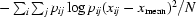; (c) homogeneity,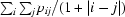; (d) energy,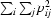; (e) contrast,; (f) root-mean-square deviation (r.m.s.d),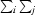.

#### 2.5. Classification schemes

For training of the neural net to proceed, a hand classification of a visually annotated set of images must be performed which samples as much of image space' as possible. In its first implementation, CEEP provides a simple classification scheme denoted by six numbers ranging from 0 (experimental mistake) to 5 (a mountable crystal) (Fig. 7).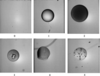Figure 7 The simple classification scheme. 0, experimental mistake, unable to find drop; 1, clear drop; 2, homogenous precipitant; 3, inhomogenous precipitant, jellyfish-like structures; 4, microcrystals or any crystals deemed as too small/bad to mount; 5, mountable (good crystals).

#### 2.6. Neural nets in CEEP

##### 2.6.1. Training of neural net

Kohonen or self-organizing neural nets have been used extensively in the analysis of multidimensional spaces (Kohonen, 1982). They are unsupervised learning systems capable of reducing multi-dimensional data to two dimensions while preserving the topology between vectors. In CEEP, a 50 × 50 node neural net, each node n = (xy), is trained with an initial set of m input patterns pmi, (where m is the pattern number, i is the number of features used) forming the feature vector. Thus each node n has a vector of weights wxy associated with it, the final net being a three-dimensional matrix of weights wxyi. The net is initiated with random weights between 0.0 and the maximum for the feature in the training set and the initial neighborhood set to 25 nodes, or half the dimension of the net. For each of 10 000 iterations each feature vector is presented to the neural net and the winning node (Wn) determined. Wn is defined as the node with the lowest Euclidean distance (Kohonen, 1982) between the pattern and the vector representing the weights of the neural net.Thus dn is the distance between node n and pattern m.

The net is then trained around the neighbourhood of the winning node Wn to respond to this pattern using a simple top-hat function such that the weights for node n in the neighbourhood of Wn are adjusted to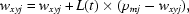where wxyj is the jth weight for node xy, L(t) is the learning rate for iteration t and pmj is the value for the jth component of pattern m.

The learning rate L(t) and neighborhood N(t) of the net is decreased according to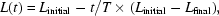where Linitial and Lfinal are the starting and final learning rates, T is the final number of iterations and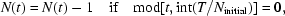where Ninitial is the starting neighborhood size, such that the final learning rate (Lfinal) and neighborhood (Nfinal) are 0.01 and 0, respectively.

Using this strategy, the net slowly converges by clustering like patterns together (Fig. 8). Finally, the reverse procedure is carried out and each node is presented to each of the feature vectors and the lowest Euclidean distance calculated. Using this method, each node is assigned the annotation value (between 1 and 5) of the best matching pattern (Fig. 8) and the trained neural net and classification map are then output to be used in future classifications. Depending on the number of patterns, the size of the net and the number of features in the feature vector, training can take several hours to calculate the net and classification map required for classification. Currently, the training set consists of 620 hand-classified images with approximately the same numbers of images contributing to each class from 1 to 5.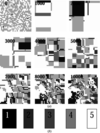Figure 8 (a) Images of neural net taken at various stages of training, starting at the 0th iteration with random weights and a neighborhood of 25 to the 10 000th iteration, a trained net with a neighborhood of 0. (b) Grayscale showing the classification number corresponding to the gray level of the node.
##### 2.6.2. Classification

Classification of a new image proceeds by extracting the appropriate features from the image and finding the winning node, defined as the lowest Euclidian distance between node and feature vector, from the pre-trained neural net (Figs. 1and 8; §2.6.1). This node then maps directly to the stored class map. Annotations are then output along with a confidence of correctness as defined by the percentage of images of one type landing on that node and the values of all of the parameters. All parameters and annotations are stored in a MySQL database administered by Perl scripts for easy retrieval and analysis of the data.

##### 2.6.3. Confidence of correctness

A simple statistic is used to estimate how likely a classification is to be correct. The class map has 2500 nodes and each of these contains information of how many images of a particular type land on them from the training set. The confidence of correctness is thus defined as the percentage of images of a particular type that land on that particular node. Thus, if an image lands on the node where all clear drop images lie, then it is highly likely that this image is also a clear drop. Those nodes without any training images landing on them are still classified, but are given an undefined confidence of correctness.

##### 2.6.4. Updating the training set

Even with the 6–11 parameters of image space currently defined, the space of the feature vector is very large. Classification should become more accurate as this feature vector space is fleshed out' with adequate sampling of the space. Selection for further inclusion into the training set is carried out semi-automatically by extracting all images that have an undefined' confidence of correctness, meaning they do not land on a node occupied by images in the training set. These images are inspected visually and classified for future inclusion into training sets.

#### 2.7. Time series of images

It is apparent that crystallogenesis is a growth phenomena. Crystals appear (and sometimes disappear) and grow over time. Other phenomena found in drops tend to be unchanging with time; precipitants tend to develop over minutes rather than days. Thus, by taking an image of a drop as a time zero' soon after the drop is set up and examining the changes in the drop over time, useful information may be derived. A simple application of this is to look at the change in the node that an image lands on, For example, the image from Fig. 9(a) lands on node (8, 18) of the neural net and is classified incorrectly' as a 3 (inhomogeneous precipitant). After the drop is analyzed a week later, the image (Fig. 9b) lands on node (27, 21) and is correctly classified as a 5 (mountable crystal). The important understanding here is that the net recognizes that the image has changed, indicating that what is in the drop may be interesting.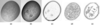Figure 9 An example of image registration. (a) Image taken at time 0 (2–4 h after setup) clearly showing precipitant. (b) Drop after one week; four crystals are apparent in the precipitant. (c) Images (a) and (b) registered, maximizing correlation coefficient to 75%, subtracted and scaled using a local scaling algorithm with a box size of 15 pixels. (d) Edges detected using image (b). (e) Edges detected using image (c), showing the increase in the signal to noise after image subtraction takes place.
##### 2.7.1. Image registration

To further analyze a time series of images, the images must be brought into register with one another to gain maximum signal to noise; this can be performed by maximizing the correlation between the two images. The calculation can be performed in real or reciprocal space. In real space, rather than maximizing a pixel-by-pixel correlation, which would be computationally too expensive, the sums of the grayscale values along each pixel row and column are calculated. The overlap of these is then maximized by two one-dimensional correlation calculations. The reciprocal-space evaluation involves computing the Fourier transformation of both images, followed by a calculation of a correlation via a fast Fourier transform. The registered images may then be scaled relative to each other and subtracted. Experiments have been conducted using a global scaling algorithm using only one scale or locally scaling using a scale derived from a window around each pixel. The local scaling algorithm calculates scales over windows of 15–30 pixels and is more computationally expensive, but generally leads to smoother and better resultant images (Fig. 9).

Fig. 9shows an image containing precipitant (Fig. 9a) and precipitant plus crystals (Fig. 9b). Upon application of this method the precipitant is mostly removed (Fig. 9c). Of course, this algorithm will only enhance the signal in crystal detection and classification of precipitants must be performed on individual images or as a consensus score from a group of images.

### 3. Results and discussion

As part of the Joint Center for Structural Genomics (Lesley et al., 2002), the entire proteome of Thermotoga maritima has been processed for expression and crystallization. Those proteins that expressed in milligram quantities were robotically set up in sitting-drop vapor-diffusion crystallization trials at 293 K with 480 conditions and a total drop volume of 100 nl. Images were taken between 2 and 4 h after setup and at 7 and 28 d intervals. Currently, 556 518 images have been processed with CEEP using the edge-detection parameters, taking approximately 1 s per image on a single-processor (R12000) Silicon Graphics Octane computer. A limited subset, mostly picked crystals, was also subjected to edge- and texture-analysis parameters with a distance of one pixel and five isotropic texture parameters taking approximately 10 s. Fig. 10illustrates the partition of all of the images into the various categories: 39.4% are classified as clear drops, 21.7% as homogeneous precipitants and 13.1% as inhomogeneous precipitants. Throughout the process between 15 and 25% of images were identified as interesting' (designated 4 or 5).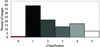Figure 10 Histogram of the classification of the 565 200 images into different categories. The shading of histogram corresponds to the shading in Fig. 8, with the exception of class 0 (experimental mistakes) which are shaded in red.

In addition to this, the images were hand analyzed looking only for crystals (categories 4 and 5). Of 920 images identified as possible crystals by humans, 72% were accurately identified as crystals using the six edge-detection parameters described (Table 2), whilst 75% were classified as crystals using the 11 parameters of the edge plus texture map. Of the other categories (1–3), from a limited set of 300 of each category of classified images, clear drops were positively identified 82% of the time, homogeneous precipitants 55% of the time and inhomogeneous precipitate 47% of the time using only the six edge-parameter neural net.

 Table 2Results on images classified by eye as mountable crystals
 Summary of results calculated on identified crystals with two self-organizing neural nets calculated with six edge parameters and 11 edge plus texture parameters (isotropic directions pixel window size of 1). Class 0 is not classified by neural net but by the drop-finding procedure (see §2.2). A false negative is defined as a crystal image which is scored below category 4.
Neural net/computed classification 0 (%) (No.) 1 (%) (No.) 2 (%) (No.) 3 (%) (No.) 4 (%) (No.) 5 (%) (No.) Crystal 4 + 5 (%) (No.) False negative (%) (No.)
Edge map, six parameters 0 (0) 3.36 (31) 8.4 (77) 15.2 (140) 37.5 (345) 35.5 (327) 73.0 (672) 26.9 (248)
Edge + text map, 11 parameters 0 (0) 5.5 (51) 3.2 (30) 15 (138) 32.5 (299) 43.2 (397) 75.6 (696) 23.8 (219)

#### 3.1. Edge detection

In general, problems are posed by large gradients on an image that do not arise from crystals (Fig. 11). Because the crystallization drops bead up and form a lens-like structure, the outside edges tend to darken (see Fig. 2b). Foreign bodies (fibers, dust, etc.) in the drops also tend to be flagged as crystals by the program. Protein skins' which buckle as the drop volume decreases also give rise to lines that look much like the edges of crystals. Inhomogeneous precipitates, generally formed by precipitation of protein immediately upon mixing with the reservoir solution, lead to sharp regular boundaries usually interpreted as crystal edges. Scratches introduced on the plastic shelf where the drop sits, either during manufacture or handling (e.g. pipetting), are usually flagged as possible crystals. On the other hand, very thin plate-like crystals are generally missing one or more edges, giving them low scores. Drops with extremely low contrast edges, e.g. those employing organic solvents, are sometimes not found at all (Fig. 11).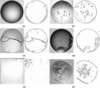Figure 11 Problem images. (a) Gradient-edge effects producing edges near the boundary of the drop; (b) drop shadows; (c) artifacts in the drops; (d) protein skins mimicking crystals; (e) very faint drop boundaries from organic precipitants; (f) inhomogeneous precipitants often look interesting to the net and generate a lot of false positives.

In general, the thresholds have been set low enough that when the program errs, it errs on the side of caution by flagging things that might be crystals (to be checked by visual inspection later) rather than missing evidence of a crystal altogether. Therefore, the program intentionally produces more false positives than false negatives.

#### 3.2. Textures

As would be expected, the evaluation of global parameters on drops can be heavily influenced by the strongest features in the image, notably the drop edges. However, if the training set of images from a wide range of different crystallization conditions is sampled well enough, this effect can be overcome. The detection process using texture only slightly enhanced crystal detection but greatly increased the computational expense. This enhancement probably arose from the detection of correlations in intensity arising from crystal edges. It does seem likely that the texture functions will be more effective in the identification and classification of precipitants and their differentiation from crystals.

#### 3.3. Classification

With only six classification categories, the scheme grossly over-simplifies the vast number of different drop conditions produced by crystallization screens. Many categories have been omitted for simplicity, such as the overlapping categories that occur in many drops, i.e. precipitant plus crystals. In these cases, correct classification is compromised and false negatives can result if one class is dominant. Further, many commonly occurring classes such as protein skins are omitted altogether. Fortunately, such classes are often marked as false positives and as such can be further eliminated by visual inspection.

### 4. Further directions/approaches

Mapping image space more completely with a better-sampled and more complete training set should provide an improved classification. Time-series information may also increase the accuracy of crystal detection by removing unchanging phenomena in the drop and increasing the signal to noise. In addition, other parameters such as those provided by multi-scale techniques for image analysis are under investigation to complement or replace those already available.

### 5. Summary

This method of image analysis provides a quick and convenient approach to filtering and annotating images taken in a robotic crystallization environment. False-positive generation is currently a major problem, with approximately 25% of images being marked as crystalline and needing user attention. The low correct scores for both precipitant categories primarily arise from their elevation to crystalline categories. Markedly more troubling for the crystallographer is any degree of false negatives (missed crystals) evaluated by the program. This will be a recurrent problem in automated crystal detection, primarily owing to the low signal to noise in many of the images and the inability of many of the features to accurately describe what is a crystal. However, the current implementation of CEEP is fast, accurate and allows the classification of up to 90 000 images per day using only a moderately powerful CPU.

Although the analysis of crystallization images by computer is by no means a substitute for a trained human, the structural genomics age approaches and the ability to process whole genomes for analysis for X-ray crystallography by robotic means must be complemented by the computational means to track and evaluate all of the processes downstream of this.

### 6. Availability

The CEEP package is written in C and has currently been tested on Silicon Graphics Octane computers, Linux running on a PC and Linux running on Dec ALPHA processors. It is available upon request from the author for non-commercial use.

### Acknowledgements

The authors gratefully acknowledge Dr Mike Heath (University of South Florida) for allowing them to use his Canny edge-detection software as the basis of their own edge detection, Peter Schultz for continuing support throughout the project, Bruce Nicholson, Ray Stevens and Dan Vaughn at Syrrx Inc., San Diego for discussion and useful images, Michael Wahl of Veeco-Optimag for valuable assistance with the Optimag 1700 and the shelf-detection software, Chris Lee for useful discussion and Teresa Lesley and Alyssa Robb for the painstaking manual classification of images.

### ReferencesBIOLOGICALCRYSTALLOGRAPHY